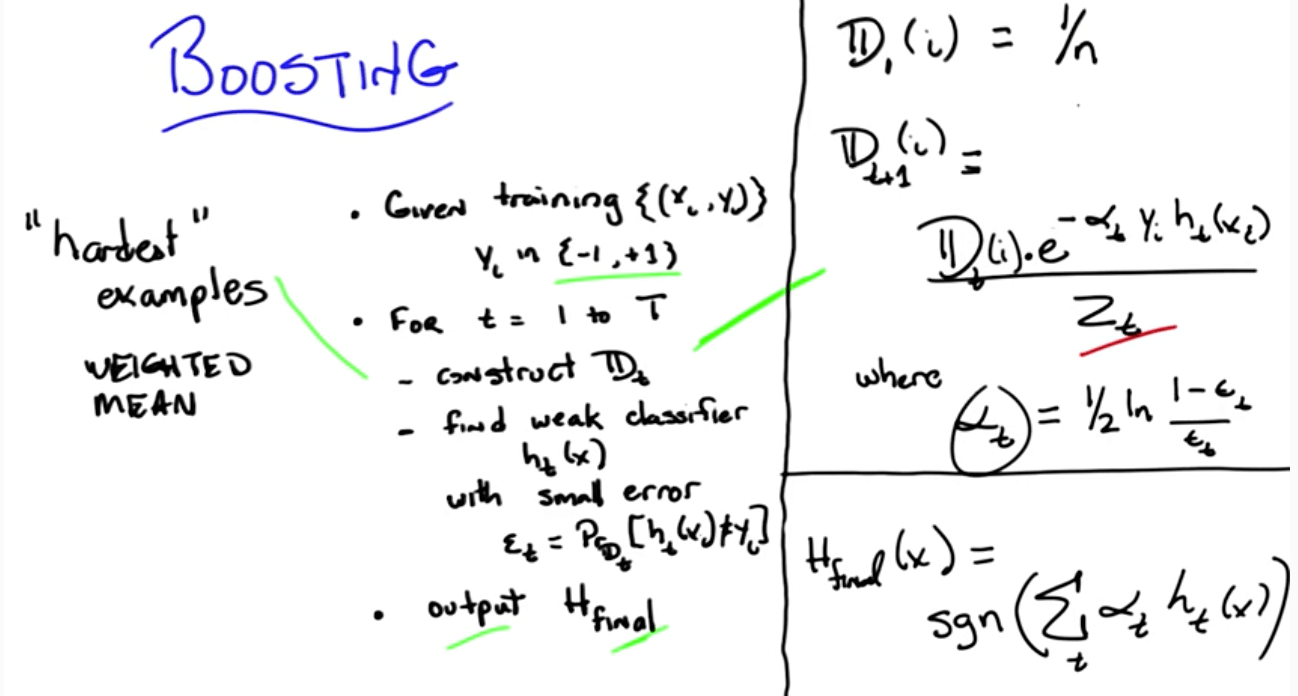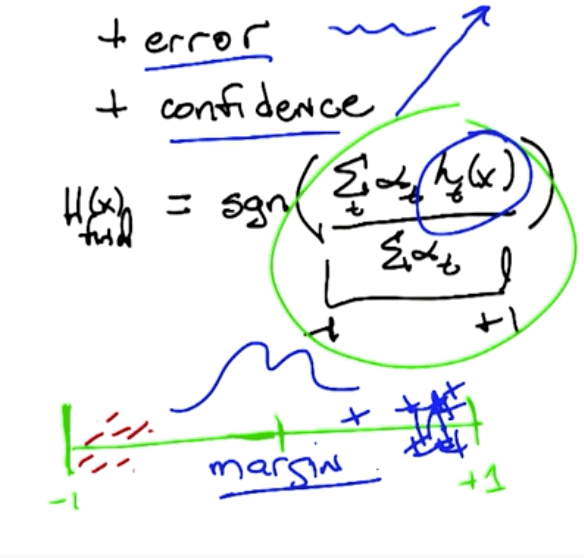Bagging, boostrap aggregation, boosting, and adaboost as a boosting method.

## Ensemble Learning: Boosting¶

I would like to give full credits to the respective authors as these are my personal python notebooks taken from deep learning courses from Andrew Ng, Data School and Udemy :) This is a simple python notebook hosted generously through Github Pages that is on my main personal notes repository on https://github.com/ritchieng/ritchieng.github.io. They are meant for my personal review but I have open-source my repository of personal notes as a lot of people found it useful.

Characteristics of Ensemble Learning

• Intuition
• You take a bunch of simple rules
• On their own, they do not give good answers
• But once you take them together, they do something good

Bagging or Bootstrap Aggregation

• Algorithm
1. Learn over a subset of data to come up rules
• Uniformly and randomly choose the data
• Apply a learning algorithm to get a rule (hypothesis)
2. Combine rules
• Mean
• Example
1. Say you've subsets of third order polynomials (rules)
2. You average the subsets of third order polynomials
• You're actually averaging the variances and differences
• It's good at getting rid of overfitting

Boosting

• "Superior" to Bagging
• Algorithm Intuition
1. Learn over a subset of data to come up rules
• Focus on the hardest examples to classify
2. Combine rules
• Weighted mean
• Error: probability of mismatches
• $$P_D[h(x)≠c(x)]$$
• D: distribution
• h(x): specific hypothesis; concept we think is right
• c(x): true underlying concept
• Weak learner: learner that does better than chance
• $$P_D[h(x)≠c(x)] ≤ \frac{1}{2} - ε$$
• Please note the difference between hypothesis and learning model, the learning model is able to choose different hypothesis according to the distribution, only if we cannot find any hypothesis that can do better than chance, then the learning model is not a weak learner
• Algorithm Overview
•• There will be more weight for the ones that the algorithms got wrong (harder examples)
• There will be less weight for the ones that the algorithms got correct (easier examples)

Boosting Benefits

1. The more hypotheses you add, the greater the margin for error
• Less overfitting
•2. Agnostic to any learner
• So long it's a weak learner

Boosting tends to overfit:

• If weak learner uses artificial neural network (ANN) with many layers and nodes
• If underlying weak learner is already overfitting
• If there's pink noise
• White noise: gaussian

• Can be used for both regression and classification problems

Explanation from scikit-learn

• The core principle of AdaBoost is to fit a sequence of weak learners (i.e., models that are only slightly better than random guessing, such as small decision trees) on repeatedly modified versions of the data
• The predictions from all of them are then combined through a weighted majority vote (or sum) to produce the final prediction
• The data modifications at each so-called boosting iteration consist of applying weights w_1, w_2, ..., w_N to each of the training samples
• Initially, those weights are all set to w_i = 1/N, so that the first step simply trains a weak learner on the original data
• For each successive iteration, the sample weights are individually modified and the learning algorithm is reapplied to the reweighted data
• At a given step, those training examples that were incorrectly predicted by the boosted model induced at the previous step have their weights increased, whereas the weights are decreased for those that were predicted correctly
• As iterations proceed, examples that are difficult to predict receive ever-increasing influence
• Each subsequent weak learner is thereby forced to concentrate on the examples that are missed by the previous ones in the sequence
In :
from sklearn.metrics import accuracy_score
from sklearn.cross_validation import train_test_split

In [ ]:
# Create data
X = iris.data
y = iris.target
X_train, X_test, y_train, y_test = train_test_split(X, y, test_size=0.2, random_state=10)

In :
# Instantiate

# Fit
abc.fit(X_train, y_train)

# Predict
y_pred = abc.predict(X_test)

# Accuracy
accuracy_score(y_pred, y_test)

Out:
0.96666666666666667

Parameter Tuning with GridSearchCV

• The main parameters to tune to obtain good results are
• n_estimators
• complexity of the base estimators
• e.g., its depth max_depth or minimum required number of samples at a leaf min_samples_leaf in case of decision trees
Tags: